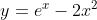## Inflections

For the curve represented by the equation below, find the point of inflection(s).Hint
First, find the second derivative of $$f(x)$$ .
Hint 2
Set $$f''(x)=0$$ to solve for the inflection point.
An inflection point is a point on the curve/graph at which concavity changes, and occurs when $$f''(x)=0$$ .
Using the power rule for the first derivative and applying it twice, we’ll get the second derivative power rule:
$$\frac{d^2}{dx^2}[x^n]=\frac{d}{dx}\frac{d}{dx}[x^n]=\frac{d}{dx}[nx^{n-1}]=n\frac{d}{dx}[x^{n-1}]=n(n-1)(x^{n-2})$$$Thus, the second derivative is: $$f''(x)=e^x-2(2)(2-1)(x^{2-2})$$$
$$=e^x-(4)(1)(x^{0})$$$$$f''(x)=e^x-(4)(1)(1)=e^x+4$$$
Note that $$\frac{d(e^u)}{dx}=e^u\frac{du}{dx}$$ , which is why $$e^x$$ remains unchanged after performing the second derivative. Solving for $$x$$ when $$f''(x)=0$$ to get the x-component inflection point:
$$e^x-4=0$$$$$ln(e^x)=ln(4)$$$
$$x=1.39$$$The inflection point consists of both a $$x$$ and $$y$$ coordinate. We have solved the $$x$$ component, but still need to determine the $$y$$ component. To find $$y$$ , plug the $$x$$ component back into the original function: $$y=e^{1.39}-2(1.39)^2$$$
$$y=4.01-2(1.93)=4.01-3.86=0.15$$\$
Thus, the inflection point is at (1.39, 0.15)
(1.39, 0.15)